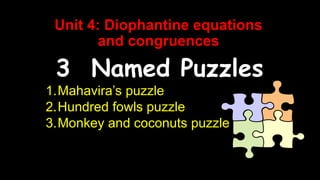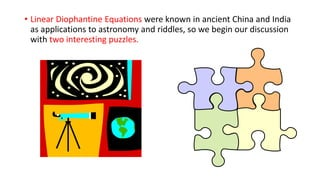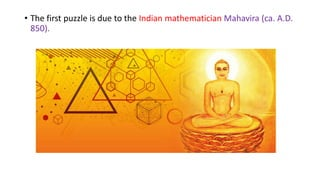Successfully reported this slideshow.

# diophantine equation and congruence puzzles×

# diophantine equation and congruence puzzles

Mahavira’s puzzle
Hundred fowls puzzle
Monkey and coconuts puzzle

Mahavira’s puzzle
Hundred fowls puzzle
Monkey and coconuts puzzle

### diophantine equation and congruence puzzles

1. 1. Unit 4: Diophantine equations and congruences 3 Named Puzzles 1.Mahavira’s puzzle 2.Hundred fowls puzzle 3.Monkey and coconuts puzzle
2. 2. • Linear Diophantine Equations were known in ancient China and India as applications to astronomy and riddles, so we begin our discussion with two interesting puzzles.
3. 3. • The first puzzle is due to the Indian mathematician Mahavira (ca. A.D. 850).
4. 4. • Mahavira, an astronomer and mathematician at the court of King Amoghavardana Vripatunga (814–877), was born in Mysore, India. • Only little is known about his life. A staunch Jain by religion, he is known for his Ganita-Sara- Sangraha, the most scholarly treatise of the time on Indian mathematics. • Written in nine chapters, it summarizes the body of knowledge then known in India, in arithmetic, including zero, fractions, and the decimal system, and geometry. It was translated from Sanskrit into English in 1912.
5. 5. • 23 weary travelers entered the outskirts of a lush and beautiful forest. • They found 63 equal heaps of plantains and seven single fruits, and divided them equally. • Find the number of fruits in each heap.
6. 6. • Let x denote the number of plantains in a heap and y the number of plantains received by a traveler. • Then the total number of plantains = 63x+7 • Each traveler received y plantain • Therefore, the total number of plantains received by the travellers =23y • Then we get the LDE 63x + 7 = 23y 63x - 23y = -7 ----------------- eqn 1
7. 7. 1. Using congruences, solve Mahavira’s puzzle We have 63x−23y=−7. This LDE creates two linear congruences: 63x≡−7 (mod 23) −23y≡−7 (mod 63) We solve 63x≡−7 (mod 23) Since 63≡−6 (mod 23) we get -6x≡−7 (mod 23) => 6x≡7 (mod 23) Here a=6 b=7 m=23 Gcd of (a,m )where (6, 23) = 1. => d =1 We find x0 =5 is a particular solution since 30≡7 (mod 23) The general solution is x=x0+(m/d)t =>5+23t where t belongs to Z
8. 8. 1. So the general solution of the congruence 63x≡−7 (mod 23) is x = 5+23t. 2. Substitute for x in the LDE and solve for y: 3. 63(5+ 23t)−23y=−7 4. 315+ 1449t −23y=−7 5. y = 14+63t 6. Thus, the general solution of the LDE is x = 5+23t, y = 14+63t, with t an arbitrary integer.
9. 9. • Another ancient riddle, called the hundred fowls puzzle, is found in the Mathematical Classic, a book by the sixth-century Chinese mathematician Chang Chiuchien.
10. 10. If a cock is worth five coins, a hen three coins, and three chicks together one coin, how many cocks, hens, and chicks, totaling 100, can be bought for 100 coins?
11. 11. Let x, y, and z denote the number of cocks, the number of hens, and the number of chicks respectively. Clearly, x, y, z ≥ 0. Then the given data yield two LDEs:
12. 12. y= 100 – 7x = 25-7/4x 4 y be an integer => -7/4x must be an integer but 4 / 7 So x=4t where t is an arbitrary integer Then y=25-7t and z=75+3t Hence every solution to the puzzle is of the form x=4t, y=25-7t, z=75+3t where t is an arbitrary integer. Now to find the actual possible solutions Since x>0, t>0 and y>0, 25-7t > 0 ie., t < 25/7 so t<3 Since z>0.75+3t >0 so t > -25 so this gives us an additional information So 0<t<3 |
13. 13. Hence the riddle has four possible solutions If t=0 x=0 y=25 z=75 If t=1 x=4 y=18 z=78 If t=2 x=8 y=11 z=81 If t=3 x=12 y=4 z=84
14. 14. • The October 9, 1926, issue of The Saturday Evening Post carried a fascinating puzzle by Ben AmesWilliams, titled Coconuts. It concerned a building contractor desperate to prevent a tough competitor from getting a lucrative contract. A shrewd employee of the contractor, knowing their competitor’s love for recreational mathematics, gave him a problem so intriguing that he became obsessed with solving it and forgot to enter his bid before the deadline.
15. 15. • Five sailors and a monkey are marooned on a desert island. • During the day they gather coconuts for food. • They decide to divide them up in the morning, but first they retire for the night. • While the others sleep, one sailor gets up and divides them into five equal piles, with one left over, which he throws out for the monkey. • He hides his share, puts the remaining coconuts together, and goes back to sleep. • Later a second sailor gets up, divides the pile into five equal shares with one coconut left over, which he discards for the monkey. • One by one the remaining sailors repeat the process. In the morning, they divide the pile equally among them with one coconut left over, which they throw out for the monkey. • Find the smallest possible number of coconuts in the original pile.
16. 16. 1. Let ‘n’ be the number of coconuts 2. After 1st person remaining coconuts is , 4/5(n-1) 3. After 2nd person remaining coconuts is , 4/5(4/5(n-1)-1) 4. After 3rd person remaining coconuts is, 4/5( 4/5(4/5(n-1)-1)-1) 5. After 4th person remaining coconuts is, 4/5(4/5( 4/5(4/5(n-1)-1)-1)-1) 6. After 5th person remaining coconuts is, 4/5(4/5(4/5( 4/5(4/5(n-1)-1)-1)-1)-1)
17. 17. • The remaining coconuts is divided into 5
18. 18. Since 45, 56 are relatively prime. e.,(45, 56) = 1, so n+4 ≡ 0 (mod 56). Thus, for n to be a minimum, n+4 = 56 (56 =15625) so n = 15,621